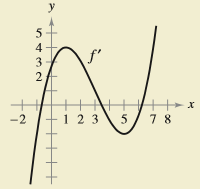Chapter 4.1, Problem 52E

Chapter
Section
Textbook Problem

HOW DO YOU SEE IT? Use the graph of f’ shown in the figure to answer the following.(a) Approximate the slope of f at x = 4 . Explain.(b) Is f ( 5 ) − f ( 4 ) > 0 ? Explain.(c) Approximate the value of x where f is maximum Explain.(d) Approximate any open intervals on which the graph of f is concave upward and any open intervals on which it is concave downward. Approximate the coordinates of any points of inflection.

(a)

To determine
The approximate slope of function f at x=4 from the graph as shown below.

Explanation

Given:

The graph of the function f,

Please Refer the figure in the given question.

Explanation:

Since, f(x) is the slope of the function f(x)

(b)

To determine
Whether f(5)f(4)>0 from the graph as shown below.

(c)

To determine
The approximate value of x where f is maximum from the graph as shown below.

(d)

To determine
Any open intervals on which the graph of f is concave upward and any open intervals on which it is concave downward from the graph as shown below. Also, approximate the x-coordinates of any points of inflection.

Still sussing out bartleby?

Check out a sample textbook solution.

See a sample solution

The Solution to Your Study Problems

Bartleby provides explanations to thousands of textbook problems written by our experts, many with advanced degrees!

Get Started

In Exercises 1728, use the logarithm identities to obtain the missing quantity.

Finite Mathematics and Applied Calculus (MindTap Course List)

For the following scores, find the value of each expression: X 3 2 4 2 a. X b. (X)2 c. X 2 d. (X 2)

Essentials of Statistics for The Behavioral Sciences (MindTap Course List)

Insert the proper sign to replacein problems 7-14. 8.

Mathematical Applications for the Management, Life, and Social Sciences

True or False: i + j is a unit vector in the direction of 5i + 5j.

Study Guide for Stewart's Multivariable Calculus, 8th

True or False: Every series must do one of these: converge absolutely, converge conditionally, or diverge.

Study Guide for Stewart's Single Variable Calculus: Early Transcendentals, 8th